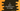# C program to print all perfect numbers in a range## C program to print all perfect numbers in a range:

In this post, we will learn how to find and print all perfect numbers in a given range. We will take the start and end values of the range from the user and print out all perfect numbers in that range.

Before that, let me quickly show you what is a perfect number and how to check if a number is perfect number or not.

### What is a perfect number and how to check if a number is perfect:

A number is called a perfect number if the sum of the divisors of that number is equal to the number itself. For example, 28 is a perfect number because its divisors are 1, 2, 4, 7, 14 and the sum of the divisors are 28. Note that we don’t have to include the number itself in the divisors list.

So, we will write one method to check if a specific number is perfect or not. And, by using a loop, it will check each values in a range if that number is perfect or not. If it will find any perfect value, it will print it.

## C program to print all perfect numbers in a range:

Let’s write down the C program that prints all perfect numbers in a given range.

``````#include <stdio.h>

int isPerfect(int n)
{
int i;
int sum = 0;

for (i = 1; i <= n / 2; i++)
{
if (n % i == 0)
{
sum += i;
}
}

if (n == sum)
{
return 1;
}
return 0;
}

int main()
{
int start, end, i;

printf("Enter the range start value: ");
scanf("%d", &start);

printf("Enter the range end value: ");
scanf("%d", &end);

printf("Perfect numbers are: \n");

for (i = start; i < end; i++)
{
if (isPerfect(i) == 1)
{
printf("%d ", i);
}
}
return 0;
}``````

Here,

• isPerfect method is used to check if a number is perfect or not. It takes one integer as the parameter and returns one integer value. If the return value is 1, it is a perfect number. If it is 0, it is not.

• Inside this method, we are using a loop from 1 to number/2 and finding out all divisors of that number.
• sum variable is used to store the sum of all divisors. This is initialized as 0.
• If it finds any divisor, it adds that to the sum variable.
• Once the loop is done, it is comparing the sum variable with the number given. If both are equal, it is a perfect number, else it is not.
• If it is a perfect number, it is returning 1. Else, it returns 0.
• Inside the main method, it is reading the start and the end values of the range and it is storing these values in the start and end values.
• By using the for loop, it is printing all perfect numbers in that range.

### Sample output:

If you run the above program, it will print output as like below:

``````Enter the range start value: 1
Enter the range end value: 100
Perfect numbers are:
6 28

Enter the range start value: 1
Enter the range end value: 1000
Perfect numbers are:
6 28 496

Enter the range start value: 1
Enter the range end value: 10000
Perfect numbers are:
6 28 496 8128``````

## C program to print all perfect numbers in a range by using a while loop:

Let’s try to find all perfect numbers in a range by using a while loop. This program is similar to the above one. The only difference is that we are using a while loop instead of a for loop to find if a perfect or not.

``````#include <stdio.h>

int isPerfect(int n)
{
int i = 1;
int sum = 0;

while (i <= n / 2)
{
if (n % i == 0)
{
sum += i;
}
i++;
}

if (n == sum)
{
return 1;
}
return 0;
}

int main()
{
int start, end, i;

printf("Enter the range start value: ");
scanf("%d", &start);

printf("Enter the range end value: ");
scanf("%d", &end);

printf("Perfect numbers are: \n");

i = start;

while (i < end)
{
if (isPerfect(i) == 1)
{
printf("%d ", i);
}
i++;
}
return 0;
}``````

Here,

• we are using two while loops.

• One is to iterate through the range of numbers and
• Another is to iterate and check if a number is perfect or not.
• All other parts of the program is same as the previous program. If you run it, it will give similar result.
``````Enter the range start value: 1
Enter the range end value: 100
Perfect numbers are:
6 28``````